# 30 Questions to test your understanding of Logistic Regression

## Introduction

Logistic Regression is likely the most commonly used algorithm for solving all classification problems. It is also one of the first methods people get their hands dirty on.

We saw the same spirit on the test we designed to assess people on Logistic Regression. More than 800 people took this test. This skill test is specially designed for you to test your knowledge on logistic regression and its nuances.

If you are one of those who missed out on this skill test, here are the questions and solutions. You missed on the real time test, but can read this article to find out how many could have answered correctly.

Here is the leaderboard for the participants who took the test.

## Overall Distribution

Below is the distribution of the scores of the participants:

##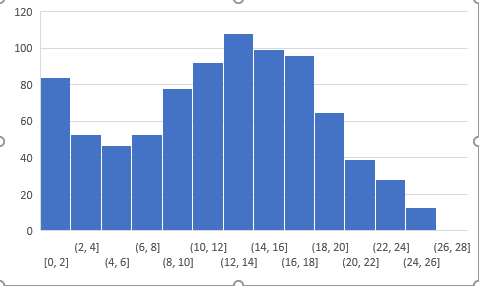You can access the scores here. More than 800 people participated in the skill test and the highest score obtained was 27.

Here are some resources to get in depth knowledge in the subject.

## Skill test Questions and Answers

1) True-False: Is Logistic regression a supervised machine learning algorithm?

A) TRUE
B) FALSE

Solution: A

True, Logistic regression is a supervised learning algorithm because it uses true labels for training. Supervised learning algorithm should have input variables (x) and an target variable (Y) when you train the model .

2) True-False: Is Logistic regression mainly used for Regression?

A) TRUE
B) FALSE

Solution: B

Logistic regression is a classification algorithm, don’t confuse with the name regression.

3) True-False: Is it possible to design a logistic regression algorithm using a Neural Network Algorithm?

A) TRUE
B) FALSE

Solution: A

True, Neural network is a is a universal approximator so it can implement linear regression algorithm.

4) True-False: Is it possible to apply a logistic regression algorithm on a 3-class Classification problem?

A) TRUE
B) FALSE

Solution: A

Yes, we can apply logistic regression on 3 classification problem, We can use One Vs all method for 3 class classification in logistic regression.

5) Which of the following methods do we use to best fit the data in Logistic Regression?

A) Least Square Error
B) Maximum Likelihood
C) Jaccard distance
D) Both A and B

Solution: B

Logistic regression uses maximum likely hood estimate for training a logistic regression.

6) Which of the following evaluation metrics can not be applied in case of logistic regression output to compare with target?

A) AUC-ROC
B) Accuracy
C) Logloss
D) Mean-Squared-Error

Solution: D

Since, Logistic Regression is a classification algorithm so it’s output can not be real time value so mean squared error can not use for evaluating it

7) One of the very good methods to analyze the performance of Logistic Regression is AIC, which is similar to R-Squared in Linear Regression. Which of the following is true about AIC?

A) We prefer a model with minimum AIC value
B) We prefer a model with maximum AIC value
C) Both but depend on the situation
D) None of these

Solution: A

We select the best model in logistic regression which can least AIC. For more information refer this source: http://www4.ncsu.edu/~shu3/Presentation/AIC.pdf

8) [True-False] Standardisation of features is required before training a Logistic Regression.

A) TRUE
B) FALSE

Solution: B

Standardization isn’t required for logistic regression. The main goal of standardizing features is to help convergence of the technique used for optimization.

9) Which of the following algorithms do we use for Variable Selection?

A) LASSO
B) Ridge
C) Both
D) None of these

Solution: A

In case of lasso we apply a absolute penality, after increasing the penality in lasso some of the coefficient of variables may become zero.

Context: 10-11

Consider a following model for logistic regression: P (y =1|x, w)= g(w0 + w1x)
where g(z) is the logistic function.

In the above equation the P (y =1|x; w) , viewed as a function of x, that we can get by changing the parameters w.

10) What would be the range of p in such case?

A) (0, inf)
B) (-inf, 0 )
C) (0, 1)
D) (-inf, inf)

Solution: C

For values of x in the range of  real number from −∞ to +∞ Logistic function will give the output between (0,1)

11) In above question what do you think which function would make p between (0,1)?

A) logistic function
B) Log likelihood function
C) Mixture of both
D) None of them

Solution: A

Explanation is same as question number 10

Context: 12-13

Suppose you train a logistic regression classifier and your hypothesis function H is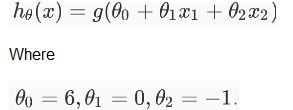12) Which of the following figure will represent the decision boundary as given by above classifier?

A)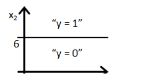B)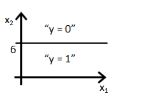C)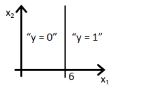D)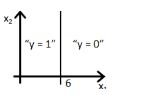Solution: B

Option B would be the right answer. Since our line will be represented by y = g(-6+x2) which is shown in the option A and option B. But option B is the right answer because when you put the value x2 = 6 in the equation then y = g(0) you will get that means y= 0.5 will be on the line, if you increase the value of x2 greater then 6 you will get negative values so output will be the region y =0.

13) If you replace coefficient of x1 with x2 what would be the output figure?

A)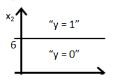B)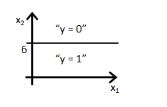C)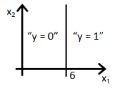D)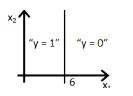Solution: D

Same explanation as in previous question.

14) Suppose you have been given a fair coin and you want to find out the odds of getting heads. Which of the following option is true for such a case?

A) odds will be 0
B) odds will be 0.5
C) odds will be 1
D) None of these

Solution: C

Odds are defined as the ratio of the probability of success and the probability of failure. So in case of fair coin probability of success is 1/2 and the probability of failure is 1/2 so odd would be 1

15) The logit function(given as l(x)) is the log of odds function. What could be the range of logit function in the domain x=[0,1]?

A) (– ∞ , ∞)
B) (0,1)
C) (0, ∞)
D) (- ∞, 0)

Solution: A

For our purposes, the odds function has the advantage of transforming the probability function, which has values from 0 to 1, into an equivalent function with values between 0 and ∞. When we take the natural log of the odds function, we get a range of values from -∞ to ∞.

16) Which of the following option is true?

A) Linear Regression errors values has to be normally distributed but in case of Logistic Regression it is not the case
B) Logistic Regression errors values has to be normally distributed but in case of Linear Regression it is not the case
C) Both Linear Regression and Logistic Regression error values have to be normally distributed
D) Both Linear Regression and Logistic Regression error values have not to be normally distributed

Solution:A

Only A is true. Refer this tutorial https://czep.net/stat/mlelr.pdf

17) Which of the following is true regarding the logistic function for any value “x”?

Note:
Logistic(x): is a logistic function of any number “x”

Logit(x): is a logit function of any number “x”

Logit_inv(x): is a inverse logit function of any number “x”

A) Logistic(x) = Logit(x)
B) Logistic(x) = Logit_inv(x)
C) Logit_inv(x) = Logit(x)
D) None of these

Solution: B

Refer this link for the solution: https://en.wikipedia.org/wiki/Logit

18) How will the bias change on using high(infinite) regularisation?

Suppose you have given the two scatter plot “a” and “b” for two classes( blue for positive and red for negative class). In scatter plot “a”, you correctly classified all data points using logistic regression ( black line is a decision boundary).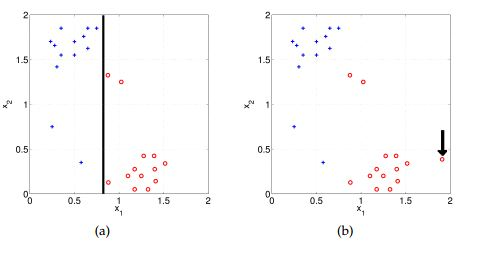A) Bias will be high
B) Bias will be low
C) Can’t say
D) None of these

Solution: A

Model will become very simple so bias will be very high.

19) Suppose, You applied a Logistic Regression model on a given data and got a training accuracy X and testing accuracy Y. Now, you want to add a few new features in the same data. Select the option(s) which is/are correct in such a case.

Note: Consider remaining parameters are same.

A) Training accuracy increases
B) Training accuracy increases or remains the same
C) Testing accuracy decreases
D) Testing accuracy increases or remains the same

Solution: A and D

Adding more features to model will increase the training accuracy because model has to consider more data to fit the logistic regression. But testing accuracy increases if feature is found to be significant

20) Choose which of the following options is true regarding One-Vs-All method in Logistic Regression.

A) We need to fit n models in n-class classification problem
B) We need to fit n-1 models to classify into n classes
C) We need to fit only 1 model to classify into n classes
D) None of these

Solution: A

If there are n classes, then n separate logistic regression has to fit, where the probability of each category is predicted over the rest of the categories combined.

21) Below are two different logistic models with different values for β0 and β1.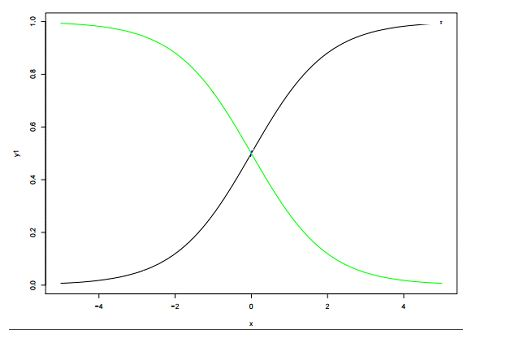Which of the following statement(s) is true about β0 and β1 values of two logistics models (Green, Black)?

Note: consider Y = β0 + β1*X. Here, β0 is intercept and β1 is coefficient.

A) β1 for Green is greater than Black
B) β1 for Green is lower than Black
C) β1 for both models is same
D) Can’t Say

Solution: B

β0 and β1: β0 = 0, β1 = 1 is in X1 color(black) and β0 = 0, β1 = −1 is in X4 color (green)

Context 22-24

Below are the three scatter plot(A,B,C left to right) and hand drawn decision boundaries for logistic regression.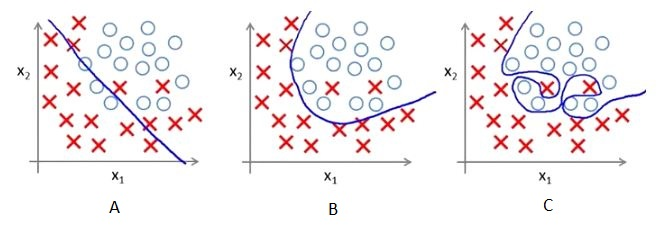22) Which of the following above figure shows that the decision boundary is overfitting the training data?

A) A
B) B
C) C
D)None of these

Solution: C

Since in figure 3, Decision boundary is not smooth that means it will over-fitting the data.

23) What do you conclude after seeing this visualization?

1. The training error in first plot is maximum as compare to second and third plot.
2. The best model for this regression problem is the last (third) plot because it has minimum training error (zero).
3. The second model is more robust than first and third because it will perform best on unseen data.
4. The third model is overfitting more as compare to first and second.
5. All will perform same because we have not seen the testing data.

A) 1 and 3
B) 1 and 3
C) 1, 3 and 4
D) 5

Solution: C

The trend in the graphs looks like a quadratic trend over independent variable X. A higher degree(Right graph) polynomial might have a very high accuracy on the train population but is expected to fail badly on test dataset. But if you see in left graph we will have training error maximum because it underfits the training data

24) Suppose, above decision boundaries were generated for the different value of regularization. Which of the above decision boundary shows the maximum regularization?

A) A
B) B
C) C
D) All have equal regularization

Solution: A

Since, more regularization means more penality means less complex decision boundry that shows in first figure A.

25) The below figure shows AUC-ROC curves for three logistic regression models. Different colors show curves for different hyper parameters values. Which of the following AUC-ROC will give best result?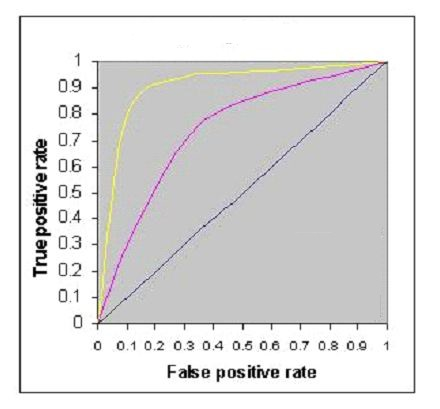A) Yellow
B) Pink
C) Black
D) All are same

Solution: A

The best classification is the largest area under the curve so yellow line has largest area under the curve.

26) What would do if you want to train logistic regression on same data that will take less time as well as give the comparatively similar accuracy(may not be same)?

Suppose you are using a Logistic Regression model on a huge dataset. One of the problem you may face on such huge data is that Logistic regression will take very long time to train.

A) Decrease the learning rate and decrease the number of iteration
B) Decrease the learning rate and increase the number of iteration
C) Increase the learning rate and increase the number of iteration
D) Increase the learning rate and decrease the number of iteration

Solution: D

If you decrease the number of iteration while training it will take less time for surly but will not give the same accuracy for getting the similar accuracy but not exact you need to increase the learning rate.

27) Which of the following image is showing the cost function for y =1.

Following is the loss function in logistic regression(Y-axis loss function and x axis log probability) for two class classification problem.

Note: Y is the target class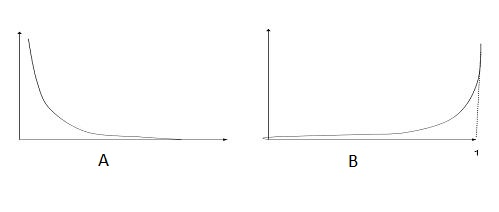A) A
B) B
C) Both
D) None of these

Solution: A

A is the true answer as loss function decreases as the log probability increases

28) Suppose, Following graph is a cost function for logistic regression.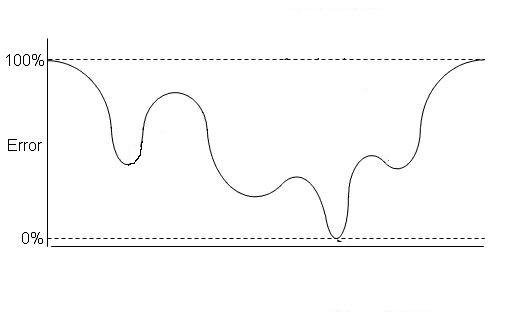Now, How many local minimas are present in the graph?

A) 1
B) 2
C) 3
D) 4

Solution: C

There are three local minima present in the graph

29) Imagine, you have given the below graph of logistic regression  which is shows the relationships between cost function and number of iteration for 3 different learning rate values (different colors are showing different curves at different learning rates ).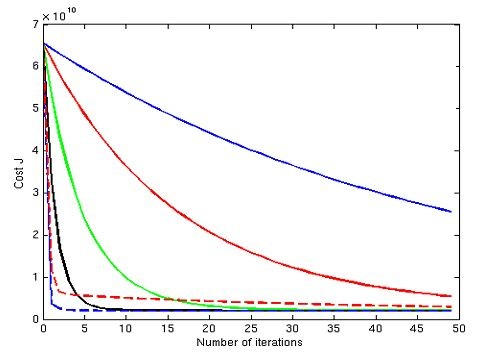Suppose, you save the graph for future reference but you forgot to save the value of different learning rates for this graph. Now, you want to find out the relation between the leaning rate values of these curve. Which of the following will be the true relation?

Note:

1. The learning rate for blue is l1
2. The learning rate for red is l2
3. The learning rate for green is l3

A) l1>l2>l3
B) l1 = l2 = l3
C) l1 < l2 < l3

D) None of these

Solution: C

If you have low learning rate means your cost function will decrease slowly but in case of large learning rate cost function will decrease very fast.

30) Can a Logistic Regression classifier do a perfect classification on the below data?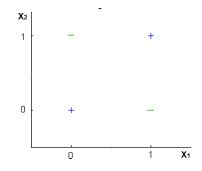Note: You can use only X1 and X2 variables where X1 and X2 can take only two binary values(0,1).

A) TRUE
B) FALSE
C) Can’t say
D) None of these

Solution: B

No, logistic regression only forms linear decision surface, but the examples in the figure are not linearly separable.

https://www.cs.cmu.edu/~tom/10701_sp11/midterm_sol.pdf

## End Notes

I tried my best to make the solutions as comprehensive as possible but if you have any questions / doubts please drop in your comments below. I would love to hear your feedback about the skill test. For more such skill tests, check out our current hackathons.

### Learn, engage,compete, and get hired!

You can also read this article on Analytics Vidhya's Android APP•Siva says:

q.19. Option A should be training accuracy increases

•Faizan Shaikh says:

Updated. Thanks for pointing it out

•agabala says:

•Faizan Shaikh says:

Hi,

You may have misunderstood the term linear separability. If the training data are linearly separable, we can select two hyperplanes in such a way that they separate the data and there are no points between them, and then try to maximize their distance. But in q30 image, it is not so.

•agabala says:

Hi Faizan,

In question 22 the data is also not linearly separable

•Ankit Gupta says:

Actually question was partially completed so we have added additional information in the note section. Thanks for pointing out

•jagan mohan says:

linear seperable in the sense

•agabala says:

q.22 shows a non-linear decision surface and q.30 states, that logistic regression has a linear decision surface. I guess, author should indicate, when exactly the decision surface is linear

•Dattatraya Rathi says:

Thank you so much sharing a good knowledge

•Ankit Gupta says:

i am glad you find them useful

•Iron Man says:

In question no. 21 you yourself proved B1 for black is greater than B1 for green. So that makes the answer to be B.

•Amit Srivastava says:

Q. 28: I can count four local minima.

•Ankit Gupta says:

you are considering global minima as local minima.

•Isma says:

You could edit the post and put the answers in the end of the post, lol

•VIVEK BARSOPIYA says:

question 20 I guess should be n-1 classifiers.
the posterior probability should sum up to one.
so if p(c_1/x)+p(c_2/x)+….p(c_k/x)=1
therfore
p(c_k/x)= 1- sum(p(c_1/x)+…..p(c_(k-1)/x)
and we need the density estimate of only k-1 classes
and the classes are assigned by
arg max p(c_i/x)
Is there something i dont know about OVA?

•Suvo Pal says:

For question no. 29 blue line (learning rate = l1) converges faster than black or any other lines.
So probable answer should be l1>l2>l3

Am i missing something here ?Enroll NowEnroll Now